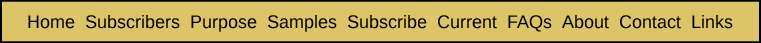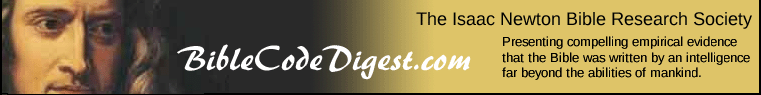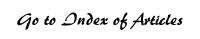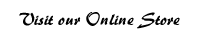Estimating the Total Number of Possible Equidistant Letter Sequences Appendix One from Bible Code Bombshell Suppose we start with a given text, such as the first 25 letters of Lincoln's Gettysburg Address. Once we remove all the spaces between its words, we have the following body of text: fourscoreandsevenyearsago We will define a letter sequence (LS) from the above text as being a grouping of consecutive letters. For example, "foursc" is an LS with six letters which begins at the start of the text. When we describe a given LS, we could describe its length in terms of the number of letters of which it consists. Alternatively, we could measure its length in terms of the number of jumps or skips between consecutive letters. For the LS, "foursc," there are five skips. First there is the one between the "f" and the "o." Second, there is the skip between the "o" and the "u." And so forth. Clearly, the number of skips in an LS is equal to the number of letters in it, less one. This gives us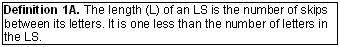Next, we will assign a number to each letter of the given text, which is its position in relation to the beginning of the text. Thus, the number 1 is assigned to the first letter in the text (f), 2 to the second (o) and so forth. The first formula we will derive is that for the number of letter sequences (LS) of a given length (L) that can be found in the text. An LS must consist of consecutive letters. We will first look only at forward LSs � those that run in the direction from the earlier to the later section of the text. Later we will consider backward LSs as well. We will denote the number of the first letter of the LS as b (for beginning) and the number of the last letter of the LS as e (for ending). The position of a given LS may then be precisely described as (b, b+1, b+2, . . ., b+L) or as (b, b+1, . . ., e). How many forward LSs of length L may be found in a text of length T? We may count the number of possible LSs by using the number corresponding with their first letter. The last LS we can form is defined by its last letter having the number e = T. That LS is then (b, b+1, b+2, . . . , T). Since this LS is also described as (b, b+1, b+2, . . . , b+L), we can equate the formulas for the position of the last letter. This gives us T = b + L. Therefore, the number of forward LSs of length L in a text of length T is T – L. This becomes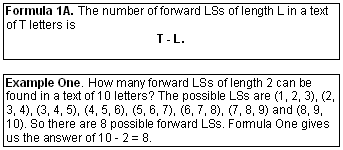It may seem inconsistent to measure the length of the text by the number of letters (T) and the length of an LS by the number of skips (L) between its letters. And it is. However, defining things this way significantly simplifies the various formulas derived in these appendices. That is why we have chosen the definitions we have presented. Let us define an equidistant letter sequence (ELS) as a letter sequence where there is a skip, or interval (i), between the successive letters in the sequence. Such a sequence would be denoted by (b, b+i, b+2i, . . ., b+L*i). An LS is a special case of an ELS where the interval i is 1. Let's take the example text above. An ELS of interval 2 beginning with the third letter in the text would be f o u r s c o r e a n d s e v e n y e a r s a g o In this example, the ELS is "usoen." As one might imagine, most ELSs formed from an intelligent text where the spaces have been eliminated will be nonsense sequences. One of our goals will be the derivation of formulas to begin to form an opinion about whether the occurrence of intelligent ELSs is a matter of coincidence or of intentional embedding within a given section of intelligent text. This will involve the deriving of formulas to determine the probability that a given ELS could appear by chance. Now "usoen" is a forward ELS because its letters progress in the same direction as the surface text. If we reversed the order of the letters in the ELS, we would have a backward ELS. In this example, it would be "neosu." How many forward ELSs of a given length L and interval i can be formed out of a text of length T? To determine this, we proceed much as we did to derive a formula for the number of possible LSs. We look at the representation of the last possible ELS: (b, b+i, b+2i, . . ., b+L*i). We know that the number of the last letter is also T, so we have the formula T = b+L*i. As in the case of the number of possible LSs, the number of possible ELSs with a given interval is the b of the last possible LS. In other words, we may start counting the possible ELSs starting with the one that begins with the first letter in the text. Then, to solve for the number of possible forward ELSs, we only need to solve the above formula for b for the last, or rightmost ELS: b = T – L*i. This gives us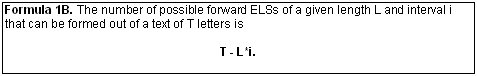Notice that when i = 1, the number of ELSs is T – L, which is Formula 1A.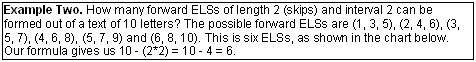Table App1AIn Table App1A, we can see that the possible forward ELSs with two skips of interval 2 which can be formed from the first ten letters in the Gettysburg Address are: `fus orc uso rcr soe cra` Let us now go on to derive a formula for the total number of possible ELSs of a given length L that can be formed out of a text of T letters when the interval can be any number between 1 and N. Suppose we set up a table (Table App1B) showing for each interval i the number of possible ELSs: Table App1B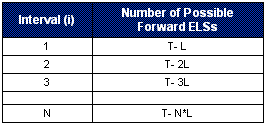There are N rows in Table App1B and the number in the second column goes down by the same amount (L) from one row to the next. The average number in the second column will be the average of the first and the last numbers � since these numbers decline by the same amount from one row to the next. That average is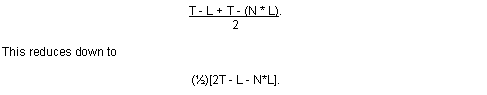The sum of the numbers in the second column will simply be the number of rows (N) times the average of the numbers in the second column. To get the number of possible ELSs, either forward or backward, we only need to multiply the above result by 2. This eliminates the (1/2), giving us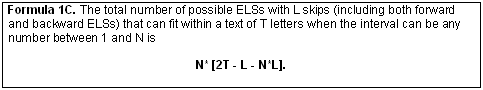Example Three: Table App1C lists every possible ELS of two skips that can be formed within a text of 10 letters. Table App1C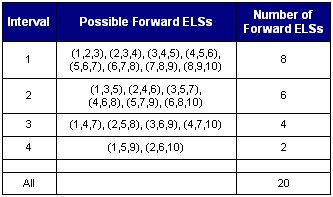Since there are 20 forward ELSs, there are also 20 backward ELSs, giving us a total of 40 possible ELSs. Formula Three also gives us 40 as a result (4 * [20 – 2 – 4*2] = 4 * 10). Suppose we want to determine the maximum number of ELSs possible from the given text, where the interval can be any possible number. To do this, we need to determine what the maximum interval M is and substitute it for N in the above formula. First, it will be helpful to develop a new concept, the span (S) of any given ELS. We will define it as the total number of spaces of text that are letters covered by or surrounded by letters in the ELS. For example, consider any of the ELSs in the last table with an interval of 1. The first one covers spaces 1, 2 and 3. Its span (S) is 3 because it occupies 3 spaces in the text. Next, take one of the ELSs with an interval of two. For example, (3, 5, 7). This ELS covers three spaces (3, 5 and 7) with its three letters. However, it also surrounds spaces four and six. So it spans five spaces (3, 4, 5, 6 and 7). Finally, let�s look at one of the ELSs with an interval of four (1, 5, 9). Obviously its span is nine (1, 2, 3, 4, 5, 6, 7, 8 and 9). Its span is again made up of the three spaces occupied by the three letters of the ELS (1, 5 and 9) and two groups of "surrounded" spaces ((2, 3, 4) and (6, 7, 8)). The number of groups of surrounded spaces will always be the same as the length of the ELS (in skips). This may be seen from Table App1D. Table App1D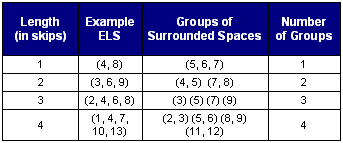This becomes apparent from examining Table App1E. Table App1E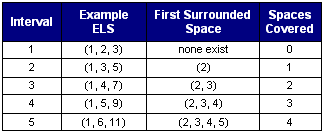In short, we have that the number of surrounded spaces spanned by a given ELS is (the # of groups of surrounded spaces) * (the # of surrounded spaces in each group of surrounded spaces) = L * (i – 1). Putting these two components together, we conclude that the total span (S) of an ELS of L letters and interval i is (the # of letters in the ELS) + (the # of surrounded spaces) = L + 1 + L * (i – 1) = L + 1 + (L * i) – L = i * L + 1. This gives usObviously, the maximum span (S) of any ELS is T. Therefore, the maximum interval (M) can be found by solving the equation T = M * L + 1. This gives us M = (T – 1)/L. However, an interval can only be a whole number. Therefore,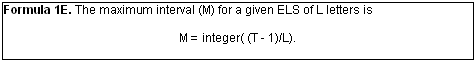In the above formula, the function "integer" means that we have deleted any numbers to the right of the decimal point. For example, integer (7.3496) = 7, and integer (3.99998) = 3, rather than 4. Since we now know what is the maximum interval possible for any ELS with L skips, we can use Formula 1C and Formula 1E to determine the total number of possible ELSs that can be formed with L skips. To do this, we only need to substitute the formula for M from Formula 1E for the variable N in Formula 1C. This gives us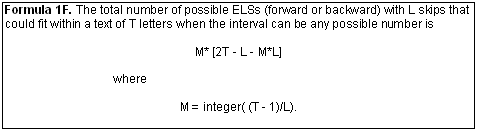Using this formula, we can quickly see what the total possible number of ELSs would be for L = 4 and different values of T � where both forward and backward ELSs are allowed. The last two values of T represent the length of the Torah (304,805) and the length of the entire Hebrew Bible (1,196,921). These are displayed in Table App1F. Table App1FWe may note that as T increases by a factor of 10 in the above table, the number of possible ELSs generally increases by a factor of 100, or 10 squared. If we were to take Formula 1F and boil it down to the terms that contribute the most to its magnitude, we would find that the total number of possible ELSs is approximately (1/L) * T^2 – T. As T becomes larger, the T^2 term is the primary contributor to the total possible number of ELSs. So, by and large, it varies largely as the square of the number of letters in the total text (T). It is also interesting to look at how the total number of possible ELSs within the Torah varies as L changes (Table App1G). Table App1GWhy does the number go down? In simple terms, the bigger something is, the fewer examples of it will fit into a given space. If we go back and look at the approximation we derived for the rough magnitude of the total number of possible ELSs, we found that it was (1/L) * T^2 – T. Since the T^2 term becomes the dominant contributor, as T becomes larger, the total number of possible ELSs will tend more and more to go down by the ratio of the successive coefficients of T^2, or by the ratio of 1/L. For example, suppose we want to approximate how much the number of possible ELSs in the Torah will decrease as L increases from 4 to 5. How much less is 1/5 than 1/4? It is 20% less, because 0.2 is only 80% of 0.25. How much less is 18.6 billion than 23.2 billion? It is 19.83%, which is very close to 20%. The title of this Appendix is, "Estimating the Total Number of Possible Equidistant Letter Sequences." Formula 1F provides that total number, once we have specified how many letters or skips are in a possible ELS. Suppose, however, that what we want to determine is the total number of possible ELSs, regardless of how short or long they are. This could be accomplished by extending the above table for skips all the way up to the maximum possible. Since the maximum skip for an ELS in the Torah is 304,804 letters, this would mean generating a table with that many rows. If there were 50 rows per page for this table, this would take nearly 6,100 pages! Intuitively, it might seem that such a number could be so large as to be infinite. However, some quick reasoning will prove otherwise. First, we note that in the above table the number of possible ELSs for a given L is always less than it was for the next smaller L. This tells us two things. The largest number of ELSs for any L is 92.9 billion. Since all of the other numbers are less than this, then the total of all of the numbers in this huge table must be less than 304,804 x 92.9 billion = 28,316,291,600,000. Although 28.3 trillion is a very large number, it is far from being "infinite." In actuality, the total number of possible ELSs that can be extracted from the Torah is only about 1.13 trillion, which is even less "infinite." How can we better estimate what the total number of possible ELSs would be? Formula 1F tells us what the number of possible ELSs is for any given L. So all we need to do is to total up those amounts for all L values from 1 to T, which is 304,805 in the case of the Torah. Mathematically, this gives us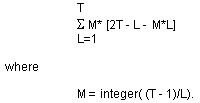First, we will use (T–1)/L as the value for M in the above formula in order to simplify the algebra. Then we will look at how much this distorts our final answer. Making that substitution, we have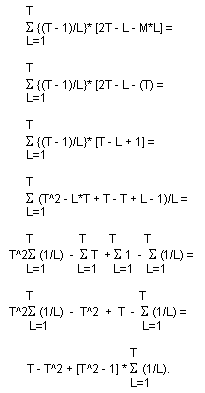For T = 304,805, the summation of values of (1/L) is equal to 13.20464, based on a full calculation of all values by a spreadsheet. If we substitute 304,805 for T in the above equation, we get that the total number of ELSs possible from the Torah is 1,133,878,222,886, or 1.13 trillion. In making the above calculation, we simplified the algebra by using (T–1)/L instead of the integer part of (T–1)/L. How much did that simplification distort the above answer? Table App1H provides a comparison of the total number of possible ELSs derived using the correct integer part of (T–1)/L and the total number obtained when the actual value of (T–1)/L is used. Table App1H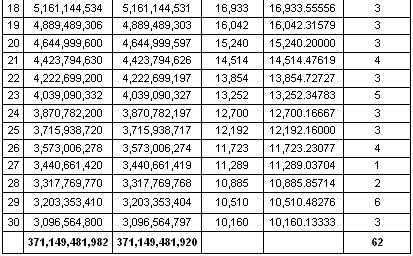As can be seen from the above comparison, the total number of ELSs for L values between 1 and 30 only differs by 62 out of 371 billion. Unfortunately, our shortcut substitution results in a somewhat lower number than the actual one. If it had resulted in a somewhat higher number, we could use the simplified calculations above to obtain a fairly tight upper bound for the total number of possible ELSs within a text with T letters. Table App1I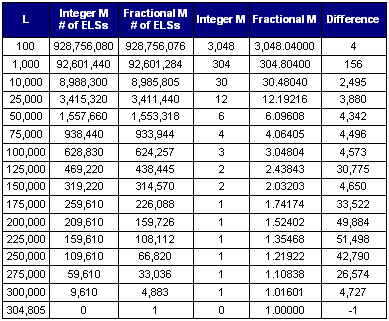In Table App1I, we look at the kinds of differences that result from a very broad range of L values � all the way up to the L value of 304,804 which is the largest value possible for the Torah. One pertinent observation is that the differences are always less than 25% of the given L value. If we assume that for every L value the differences are always less than L, we can obtain an inequality that will enable us to obtain a reasonable upper bound for the total number of possible ELSs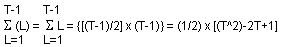since the sum of a series of integers that increase by 1 will equal the average of those integers times the number of integers being totaled. This is clearly a finite number since it is less than (1/2) x T^2. So we have that the total number of ELSs possible in a text of length T is less than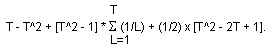This reduces down to the following inequality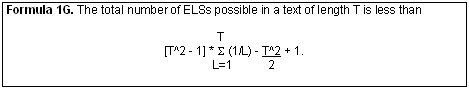This will be finite as long as the summation of values of (1/L) from L=1 to T is finite. From the book, Summation of Series, collected by L.B.W. Jolley, and published by Dover in 1961, we have from formula (70) on page 14 [as compiled from Smithsonian Mathematical Formulae (1922)] that the sum of values of (1/L) from 1 to T is equal to Euler's Constant + ln (T) + (1/(2*T)) + a series of terms with negative values. We can therefore conclude that the summation of values of (1/L) from L=1 to T is less than 0.5772156649 + ln (T) + (1/(2*T)). This means that this sum of values of (1/L) is less than 13.20464 for the Torah (where T = 304,805) and less than 14.577248 for the entire Hebrew Bible (where T = 1,196,921). It is also less than 19.17765 for 100 books which are each as long as the Hebrew Bible, and it is less than 23.78282 for 10,000 books as long as the Hebrew Bible. Using these inequalities for the values of different sums of (1/L) and different lengths of texts, we get that the total possible number of ELSs for each given text is as shown in Table App1J. Table App1JWe have generously assumed above that every book is exactly the same length as the Hebrew Bible, which is about 800 pages long, and that these books have been placed in a fixed order, so that an ELS could be formed that would start with one or more letters in one book and finish with one or more letters from a subsequent book. Since it is unlikely that a billion different books have ever been written by mankind, we may conclude that the total possible number of ELSs in all of the books that have ever been written is less than 50 followed by 30 zeros. All of these numbers, though quite large, are certainly quite finite. Therefore, it is simply not true that there are an infinite number of possible ELSs that can be discovered within either the Torah, or the Hebrew Bible or even a billion books that are each 800 pages long.

 Enjoy finding your own Bible codes. Bible code search software is available in our online store. Subscribe Free! By signing up to be a member of The Isaac Newton Bible Research Society, you will have access to more than fifteen years of research by our team of Bible code researchers. Sign up to be a member today.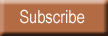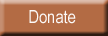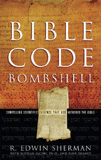Bombshell examines two massive, recently discovered clusters of codes in the Hebrew Old Testament. To read more about Bombshell, click here, or click below to order from Amazon today!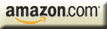| HOME | SUBSCRIBERS ONLY | OUR PURPOSE | FREE SAMPLES | CURRENT | | FAQs | ABOUT BIBLE CODES | SUBSCRIBE | CONTACT | Copyright © 2016 BibleCodeDigest.com